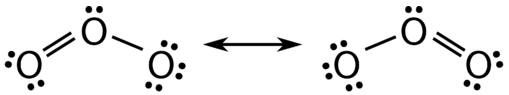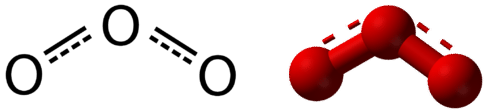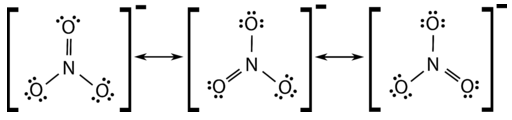# 10.6: Resonance: Equivalent Lewis Structures for the Same Molecule

Learning Objectives

• Explain the following laws within the Ideal Gas Law

## Resonance

There are some cases in which more than one viable Lewis structure can be drawn for a molecule. An example is the ozone $$\left( \ce{O_3} \right)$$ molecule in the figure below. There are a total of 18 electrons in the structure and so the following two structures are possible.Figure $$\PageIndex{2}$$: Resonance forms of ozone.

The structure on the left (see figure above) can be converted to the structure on the right by a shifting of electrons without altering the positions of the atoms.

It was once thought that the structure of a molecule such as $$\ce{O_3}$$ consisted of one single bond and one double bond which then shifted back and forth as shown above. However, further studies showed that the two bonds are identical. Any double covalent bond between two given atoms is typically shorter than a single covalent bond. Studies of the $$\ce{O_3}$$ and other similar molecules showed that the bonds were identical in length. Interestingly, the length of the bond is in between the lengths expected for an $$\ce{O-O}$$ single bond and a double bond.

Resonance is the use of two or more Lewis structures to represent the covalent bonding in a molecule. One of the valid structures is referred to as a resonance structure. It is now understood that the true structure of a molecule which displays resonance is that of an average or a hybrid of all the resonance structures. In the case of the $$\ce{O_3}$$ molecule, each of the covalent bonds between $$\ce{O}$$ atoms is best thought of as being "one and a half" bonds, as opposed to either a pure single bond or a pure double bond. This "half-bond" can be shown as a dotted line in both the Lewis structure and the molecular model (see figure below).Figure $$\PageIndex{3}$$: "Half-bond" model of ozone molecule.

Many polyatomic ions also display resonance. In some cases, the true structure may be an average of three valid resonance structures, as in the case of the nitrate ion, $$\ce{NO_3^-}$$ (see figure below).Figure $$\PageIndex{4}$$: Resonance structure of nitrate anion.

The bond lengths between the central $$\ce{N}$$ atom and each $$\ce{O}$$ atom are identical and the bonds can be approximated as being equal to one and one-third bonds.

## Summary

• Resonance structures are averages of different Lewis structure possibilities.
• Bond lengths are intermediate between covalent bonds and covalent double bonds.

## Contributors

• CK-12 Foundation by Sharon Bewick, Richard Parsons, Therese Forsythe, Shonna Robinson, and Jean Dupon.

• Henry Agnew (UC Davis)